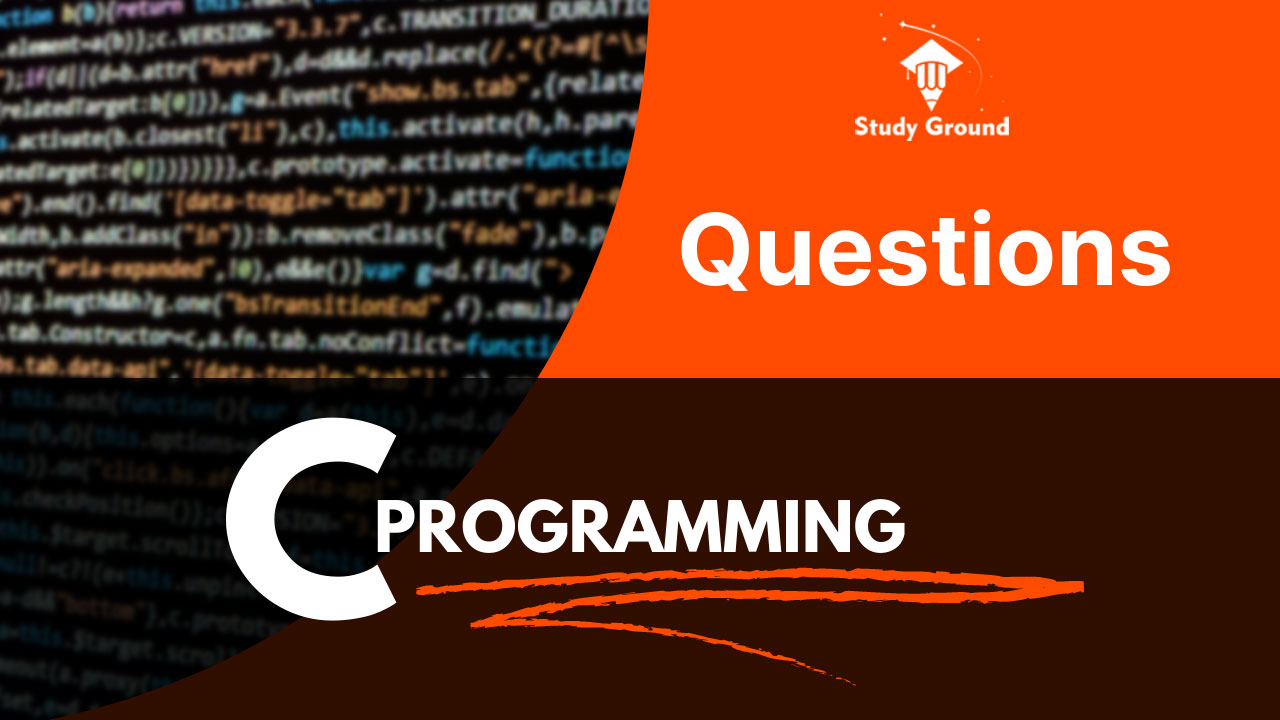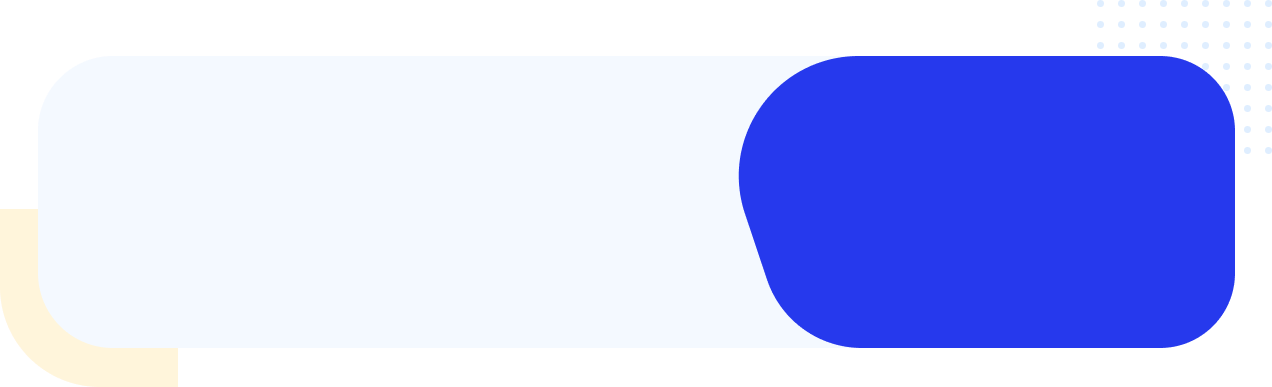# Basic Programming Questions on C Programming Language

Learning C programming provides a solid foundation in programming principles and concepts. It emphasizes procedural programming, which helps beginners understand the basics of variables, data types, loops, decision-making structures, and functions.

C programming encourages a logical and structured approach to problem-solving. As beginners tackle programming challenges in C, they learn how to break down complex problems into smaller, manageable tasks and develop algorithms to solve them efficiently.

Let us start with some basic questions to understand the basic steps of logic building.

1. Write a C program to print any message or your name
2. Write a C program to print a table like

NAME      ROLL       PHONE
ALEX      15         5415785896

3. Write a C program to input 2 numbers and perform the sum and average of integer and floating point numbers.

4. Write a C program to perform all arithmetic operations

5. Write a C program to enter a rectangle's length and breadth, calculate its area and perimeter, and give results in tabular format.

6. Write a C program to convert temperature in Celsius to Fahrenheit and vice versa.

7. Write a C program to input the base and power of a number and calculate the power of it (2^3 = 8 for example)

8. Write a C program to enter any number and calculate its square root.

9. Write a C program to calculate the area of an equilateral triangle.

10. Write a C program to enter P, T, and R  and calculate Simple Interest and Compound Interest and display the output in tabular format

.. More questions are coming soon.

Tags: Programming Exercise Basic Programming Coding Practice Programming Skills C Programming Programming Challenges Coding Practice Beginner Programming Tasks Simple Coding Exercises Fundamental Programming Problems Basic Coding Drills Programming Puzzles For Beginners Entry-level Coding Assignments Programming Exercises For Beginners Coding Problems For Novices

## Related PostsContact Now